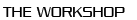﻿ 7th Workshop on Numerical Methods for Evolution Equations ::Past WorkshopsList of Talks

• Georgios Akrivis
Stability properties of implicit--explicit multistep methods for a class of nonlinear parabolic equations.
• Panagiotis Chatzipantelidis
On the preservation of positivity in some finite element methods for the heat equation.
• Konstantinos Chrysafinos
Analysis and approximations of the velocity tracking control problem for the 3D evolutionary Navier-Stokes equations.
• Alexandre Ern
Discontinuous Galerkin method in time combined with a stabilized finite element method in space for linear first-order PDEs
• Emmanuil Georgoulis
Adaptivity and blow-up detection for semilinear parabolic problems based on rigorous a posteriori bounds.
• Patrick Joly
Time domain analysis of an interface resonance phenomenon in electromagnetism.
• Theodoros Katsaounis
A posteriori error control and adaptivity for the Schrödinger equation.
• Angela Kunoth
Multilevel preconditioning for isogeometric analysis.
• Omar Lakkis
Aposteriori analysis and adaptivity in discontinuous Galerkin time-stepping methods.
• Ulrich Langer
Functional a posteriori error estimates for parabolic time-periodic problems
• Mats Larson
Stabilized cut finite element methods for evolution problems on surfaces.
Weak convergence analysis for finite element approximations of stochastic evolution PDEs.
• Christian Lubich
On backward difference formulae for quasi-linear parabolic equations.
• Babis Makridakis
Error control for wave equations.
• Alexander Ostermann
Splitting based on approximate flows.
• Andreas Prohl
Numerical discretization of the stochastic cubic Schroedinger equation.
• Kunibert Siebert
On the H1 stability of the L2 projection on adaptively refined grids
• Vanessa Styles
Multi-material structural topology optimisation using phase field methods.
• Chrysoula Tsogka
Signal to Noise: Ratio analysis in passive correlation based imaging.
• Georgios Zouraris
A numerical method for a nonlinear Fokker-Planck-Kolmogorov equation.